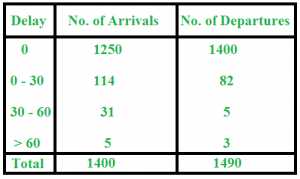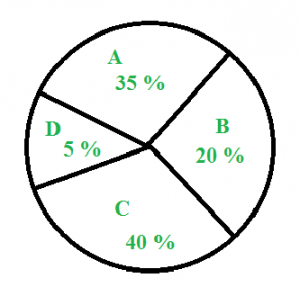GeeksforGeeks App
Open AppBrowser
Continue

# Data Interpretation – Aptitude Question and Answers

Data interpretation questions are an essential part of the Quantitative Aptitude section in various government exams, such as SSC, Bank, RRB, and Insurance exams. These questions require the ability to analyze and interpret different forms of data, including tables, graphs, charts, and more. With a focus on percentage, ratio and proportion, data interpretation questions can be challenging, making it crucial to practice and refine your skills. In this article, we will explore some commonly asked questions and provide insightful practice questions to help you enhance your data interpretation techniques.

Practice Quiz:

Practice Data Interpretation Aptitude Quiz Questions

## Sample Questions on Data Interpretation

### Q1: The following table contains the data on train arrival and train departure from a station. Study the table and answer the questions that follow :### c) Find the percentage of late-arriving trains

Solution:

a) Number of trains arriving late = 114 + 31 + 5 = 150

b) Number of trains departing late = 82 + 5 + 3 = 90

c) Percentage of late arriving trains = (150 / 1400) x 100 = 10.71 %

### c) What percent of amount on food is spent as amount on education?

Solution :

a) Food = 20 % of expenditure => Amount spent on food = 20% of 50,000 = Rs. 10,000

b) Money spent on clothing and housing together = 25 % of Rs. 50,000 = Rs. 12,500 Money spent on transportation = 20 % of Rs. 50,000 = Rs. 10,000 Therefore, more money spent on clothing and housing together than on transportation = Rs. 12,500-10,000 = Rs. 2,500

c) Expenditure on food = 20 % Expenditure on education = 5 % Therefore, percent of amount on food spent as amount on education = (5 / 20) x 100 = 25 %

### Q3: Study the pie chart below and answer the questions that follow :### c) What is the difference between the central angle of C and D ?

Solution :

a) Central angle of A = Percentage of A x 360 degrees = (35 / 100) x 360 = 126 degrees

b) Sales of B = 20 % of 1200 = 240

c) Difference between the central angle of C and D = 40 % of 360 – 5 % of 360 = 35 % of 360 = 126 degrees

### Q4. A company conducted a survey of 500 customers to determine their satisfaction with the company’s products. The results showed that 80% of customers were satisfied with the products. Of the satisfied customers, 60% were repeat customers. How many customers were repeat customers and satisfied with the products?

Solution :

To determine the number of repeat customers who were satisfied with the products, we need to first calculate the total number of satisfied customers. The survey showed that 80% of 500 customers were satisfied with the products, so the number of satisfied customers is: 80% of 500 = 0.8 * 500 = 400. Now we can find the number of repeat customers who were satisfied with the products by multiplying the total number of satisfied customers by the percentage of satisfied customers who were repeat customers: 60% of 400 = 0.6 * 400 = 240. Therefore, there were 240 repeat customers who were satisfied with the products.

Test your knowledge of Data interpretation in Quantitative Aptitude with the quiz linked below, containing numerous practice questions to help you master the topic:-

Practice Data Interpretation Aptitude Quiz Questions

## Also Practice:

My Personal Notes arrow_drop_up